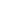FLAT 50% OFF

ENDS IN

• JEE Main
•• Mathematics
• Back to Doubt Clearing

## Solve question number 12The sum of all real values of $x$ satisfying the equation $\left(x^{2}-5 x+5\right)^{x^{2}+4 x-80}=1$ is(a) $-4$(b) 6(c) 5(d) 3

### Asked By Ankit prakash

Updated Mon, 20 May 2019 02:14 pm